.125truein

The following problems involve the PRECISE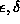DEFINITION OF LIMITS OF FUNCTIONS AS X APPROACHES A CONSTANT. Most problems are average. A few are somewhat challenging. We will begin with the precisedefinition of the limit of a function as x approaches a constant.

DEFINITION: The statementhas the following precise definition. Given any real number, there exists another real numberso that if, then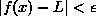.

In general, the value ofwill depend on the value of. That is, we will always begin withand then determine an appropriate corresponding value for. There are many values ofwhich work. Once you find a value that works, all smaller values ofalso work.

To try and understand the meaning behind this abstract definition, see the given diagram (to appear later). We first pick anband around the number L on the y-axis . We then determine aband around the number a on the x-axis so that for all x-values (excluding x=a ) inside theband, the corresponding y-values lie inside theband. In other words, we first pick a prescribed closeness () to L . Then we get close enough () to a so that all the corresponding y-values fall inside theband. If acan be found for each value of, then we have proven that L is the correct limit. If there is a singlefor which this process fails, then the limit L has been incorrectly computed, or the limit does not exist.

In the problems that follow, we will use this precise definition to mathematically PROVE that the limits we compute algebraically are correct. When using this definition, begin each proof by lettingbe given. Then take the expressionand, from this, attempt to algebraically ``solve for" | x - a | . At that point, an appropriate value forcan easily be determined.

The expression `` iff " will be used often in the solutions to the following problems. It means `` if and only if " or `` is equivalent to ''. The expression `` min{A, B }" will also be used in many of the solutions. It means `` the minimum value of A and B." For example, min{ 3, 7 } = 3.

PROBLEM 1: Prove that.

PROBLEM 2: Prove that.

PROBLEM 3: Prove that.

PROBLEM 4: Prove that.

PROBLEM 5: Prove that.

PROBLEM 6: Prove that.

PROBLEM 7: Prove that.

PROBLEM 8: Prove that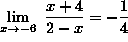.

PROBLEM 9: Prove that.

PROBLEM 10: Prove that.

PROBLEM 11: Prove that.

PROBLEM 12: Prove that.

The following two problems require some knowledge and understanding of the Mean Value Theorem.

PROBLEM 13: Prove that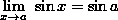, where a is any real number .

PROBLEM 14: Prove that, where a is any positive real number .

The following problem uses the triangle inequality. The triangle inequality states thatfor any two real numbers A and B .

PROBLEM 15: Let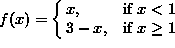. Prove thatdoes not exist .